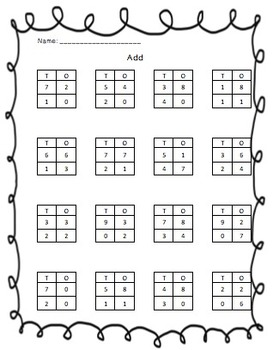Math Practice or Homework WorksheetsSubject
Resource Type
Product Rating
File Type

PDF (Acrobat) Document File

Be sure that you have an application to open this file type before downloading and/or purchasing.

762 KB|4 pages
Share
Product Description

Counting Coins
CCSS.Math.Content.1.OA.C.6 Add and subtract within 20, demonstrating fluency for addition and subtraction within 10. Use strategies such as counting on; making ten (e.g., 8 + 6 = 8 + 2 + 4 = 10 + 4 = 14); decomposing a number leading to a ten (e.g., 13 – 4 = 13 – 3 – 1 = 10 – 1 = 9); using the relationship between addition and subtraction (e.g., knowing that 8 + 4 = 12, one knows 12 – 8 = 4); and creating equivalent but easier or known sums (e.g., adding 6 + 7 by creating the known equivalent 6 + 6 + 1 = 12 + 1 = 13).

CCSS.Math.Content.1.NBT.C.4 Add within 100, including adding a two-digit number and a one-digit number, and adding a two-digit number and a multiple of 10, using concrete models or drawings and strategies based on place value, properties of operations, and/or the relationship between addition and subtraction; relate the strategy to a written method and explain the reasoning used. Understand that in adding two-digit numbers, one adds tens and tens, ones and ones; and sometimes it is necessary to compose a ten.

Ten More & Ten Less
CCSS.Math.Content.1.NBT.C.5 Given a two-digit number, mentally find 10 more or 10 less than the number, without having to count; explain the reasoning used.

Count by Tens
CCSS.Math.Content.2.NBT.A.2 Count within 1000; skip-count by 5s, 10s, and 100s
***^ Second grade standard

Comparing Coin Values
CCSS.Math.Content.1.NBT.B.3 Compare two two-digit numbers based on meanings of the tens and ones digits, recording the results of comparisons with the symbols >, =, and <.

Reading and Writing the time of an analog clock
CCSS.Math.Content.1.MD.B.3 Tell and write time in hours and half-hours using analog and digital clocks.
Total Pages
4 pages
N/A
Teaching Duration
N/A
Report this Resource to TpT
Reported resources will be reviewed by our team. Report this resource to let us know if this resource violates TpT’s content guidelines.